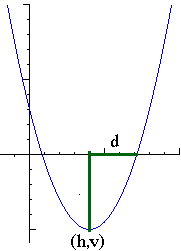by Henri Picciotto

Links to many lessons and activities, to be used in Algebra 1, Algebra 2, and with math teachers.

Multiple Representations and Connections

The Lab Gear manipulatives can help lay a foundation for work on quadratics. Manipulatives make factoring and completing the square accessible to the vast majority of students, while giving them a geometric perspective which is unfortunately missing from many curricula. That approach is all the more important if you teach this material to students in grades 8-9.

Geometry-Graphing Connection: a sample activity from my book Algebra Lab Gear: Algebra 1 (available from Didax). It makes a connection between the Lab Gear, the formulas, and the graphs of certain quadratics.

Here are some GeoGebra applets to preview, review, or discuss connections between representations — probably most helpful to students who have worked with the manipulatives, but worth checking out in either case:
Multiplying Binomials (blocks and "the box")
Squaring a Binomial
Trinomial Representations (blocks, "the box", and a graph)

An accessible approach to Completing the Square, using the Lab Gear and leading to the quadratic formula.

An apparently unrelated unit: Constant Sums, Constant Products
Images that reveal many connections, and can serve as the basis for an assessment.

These lessons involve the sparing use of graphing technology. Unlike some other tech-based approaches ("graph this, graph that, what do you notice?") this is a rather theoretical unit, which helps develop students' symbol sense and goes for more depth of understanding than is customarily expected.
Factored Form of Quadratic Functions | Applet | Geogebra file
From Factored to Standard Form
Moving Parabolas Around
Quadratic Forms (a condensed version of the above three lessons, probably better suited for teachers)
Make These Parabolas: TI-83/84, TI-89
Related GeoGebra file: Translating Parabolas
Changing a, b, c (applet: where does the vertex go when we change those parameters?)
Three Visual Paths to the Quadratic Formula

A New Path to the Quadratic Formula (Mathematics Teacher, February 2008) provides an overview of the three approaches.

1. The standard approach to the derivation of the quadratic formula (completing the square) is vastly more accessible to students who have experience with the multiple representations outlined above. (I always did a quick review of the Lab Gear representation in Algebra 2 for that reason.)

Outline of a unit: Completing the Square, including links to:
Squaring a Binomial (applet)
Completing the Square (applet)
Geometry-Graphing Connection (discussion-provoking activity)
2. A graphical derivation of the quadratic formula, based on moving a parabola:
Narrated slide show 12:12 ("Masterful" — Zalman Usiskin)
Worksheet: A Graphical Approach to the Quadratic Formula
Related GeoGebra file
3. Another graphical derivation. Late in the Math 1 / Algebra 1 course I used to teach, I asked students to create a short report explaining the connections illustrated here, using their own example. One year, while grading this assignment, it hit me that there must be a new proof of the quadratic formula based on this, and sure enough, there is. You can read it in my article in the February 2008 issue of The Mathematics Teacher, under the title "A New Path to the Quadratic Formula". The proof involves neither parabolas nor completing the square.
Background: Constant Sums, Constant Products | Connections
Preparation: Find the Dimensions
Video presentation (11:20) using this GeoGebra file
Online or Keynote presentation. Grab a pencil to follow the argument, and click your mouse to advance through the slides.
Worksheet (for teachers and perhaps some precalculus students)
Full proof: A New Path to the Quadratic Formula
Geometry of the Parabola